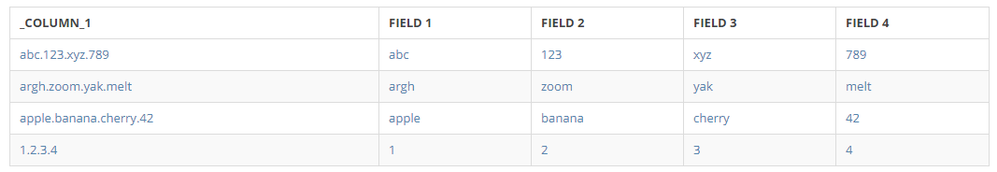# Is there a way in beast mode to split a column by a common character?

Hello,

I have a a field where the data comes in abc.123.xyz.789. My goal is to create 4 beast modes, where each beast mode will return one segment of the original field. I looked up how to do in a dataflow using substring_index, but that does not appear to be a valid function in a beast mode equation. SUBSTRING_INDEX(stringdelimiternumber)

Original field: abc.123.xyz.789

Beast mode 1 result = abc

Beast mode 2 result = 123

Beast mode 3 result = xyz

Beast mode 4 result = 789

Is this possible to do in a beast mode, or do I have to run through a dataflow to complete?

Thanks

• You would need to combine the INSTR,SUBSTRING, and LENGTH functions to do this in a beast mode.

With that being said, I STRONGLY ADVISE AGAINST DOING THIS IN A BEAST MODE.

But you asked if it was possible... and it is... but it causes what seems to be A LOT of validation time on my 4 line dataset consisting of the following values:

`abc.123.xyz.789 argh.zoom.yak.meltapple.banana.cherry.421.2.3.4`

Field 1 (seemed simple enough, lets go!)

`SUBSTRING(`_COLUMN_1`,1,INSTR(`_COLUMN_1`,'.')-1)  -- SUBSTRING(`COLUMNWITHDATA`,[STARTING POSITION],[STRING LENGTH])						   -- Starting Position = 1 (first character)                                                   -- String Length = Position of first period character                                                   -- -1 as we must consider the first period character and backup one character`

Field 2 (here's where you start to get the idea... of why this is a bad idea... I tried commenting this out to make it easier to follow... but I admit I may have failed to do so in the endeavor)

`SUBSTRING(SUBSTRING(`_COLUMN_1`,INSTR(`_COLUMN_1`,'.')+1,LENGTH(`_COLUMN_1`)-LENGTH(SUBSTRING(`_COLUMN_1`,1,INSTR(`_COLUMN_1`,'.')-1))),1,INSTR(SUBSTRING(`_COLUMN_1`,INSTR(`_COLUMN_1`,'.')+1,LENGTH(`_COLUMN_1`)-LENGTH(SUBSTRING(`_COLUMN_1`,1,INSTR(`_COLUMN_1`,'.')-1))),'.')-1)-- CONSIDER FIELD1:-- SUBSTRING(`_COLUMN_1`,1,INSTR(`_COLUMN_1`,'.')-1) -- SUBSTRING([STRING],[STARTING POSITION],[NUMBER OF CHARACTERS])												     -- Starting Position = 1 (first character)                                                     -- String Length = Position of first period character                                                     -- -1 as we must consider the first period character and backup one character-- FIELD2:-- SUBSTRING(		-- this SUBSTRING, is what I will refer to as SUBSTRING1-- first parameter of SUBSTRING1 begins:-- SUBSTRING(`_COLUMN_1`,		 -- FIRST PARAMETER of SUBSTRING2 - [STRING]='abc.123.xyz.789'-- INSTR(`_COLUMN_1`,'.')+1,	 -- SECOND PARAMETER of SUBSTRING2 Field1 add 1 (find the period, shift right one position) [STARTING POSITION]=5-- LENGTH(`_COLUMN_1`)-LENGTH(SUBSTRING(`_COLUMN_1`,1,INSTR(`_COLUMN_1`,'.')-1)) -- THIRD PARAMETER of SUBSTRING2 Find length of [STRING] and subtract the length of Field1, to return the length of the remainder of [STRING].. [NUMBER OF CHARACTERS]=11-- first parameter of SUBSTRING1 ends, so now SUBSTRING1 has [STRING]='123.xyz.789'-- second parameter for SUBSTRING1 begins:-- 1, -- We're starting at position one, of '123.xyz.789'-- third parameter of SUBSTRING1 begins-- EDITOR'S NOTE: we have to find the length of '123' but we have to find it from 'abc.123.xyz.789' because we can't store '123.xyz.789 in a variable, which would make this tremendously easier.-- INSTR(  		-- NEED TO FIND THE POSITION OF the first period in '123.xyz.789' so first we have to return 123.xyz.789 with SUBSTRING3-- SUBSTRING(`_COLUMN_1`, -- FIRST PARAMETER of SUBSTRING3 (abc.123.xyz.789)-- INSTR(`_COLUMN_1`,'.')+1, -- SECOND PARAMETER of SUBSTRING3 [STARTING POSITION]=5-- LENGTH(`_COLUMN_1`)-LENGTH(SUBSTRING(`_COLUMN_1`,1,INSTR(`_COLUMN_1`,'.')-1))) -- THIRD PARAMETER OF SUBSTRING3 [NUMBER OF CHARACTERS]=3 -- whole string length (15), minus the length of Field1 'abc' gives us 12-- ,'.') -- FIND THE POSITION of the first period in '123.xyz.789'-- -1 -- subtract one more length character, for the period delimiter gives us 11-- Third parameter of SUBSTRING1 ends [NUMBER OF CHARACTERS]=3-- ) -- END SUBSTRING1`

Field 3 (note that explanations/comments have disappeared entirely at this point...)

`SUBSTRING(	SUBSTRING(SUBSTRING(`_COLUMN_1`,INSTR(`_COLUMN_1`,'.')+1,LENGTH(`_COLUMN_1`)-LENGTH(SUBSTRING(`_COLUMN_1`,1,INSTR(`_COLUMN_1`,'.')-1))),    INSTR(SUBSTRING(`_COLUMN_1`,INSTR(`_COLUMN_1`,'.')+1,LENGTH(`_COLUMN_1`)-LENGTH(SUBSTRING(`_COLUMN_1`,1,INSTR(`_COLUMN_1`,'.')-1))),'.')+1    ,LENGTH(`_COLUMN_1`)-LENGTH(INSTR(SUBSTRING(`_COLUMN_1`,INSTR(`_COLUMN_1`,'.')+1,LENGTH(`_COLUMN_1`)-LENGTH(SUBSTRING(`_COLUMN_1`,1,INSTR(`_COLUMN_1`,'.')-1))),'.'))             )  ,1  ,INSTR(SUBSTRING(SUBSTRING(`_COLUMN_1`,INSTR(`_COLUMN_1`,'.')+1,LENGTH(`_COLUMN_1`)-LENGTH(SUBSTRING(`_COLUMN_1`,1,INSTR(`_COLUMN_1`,'.')-1))),          INSTR(SUBSTRING(`_COLUMN_1`,INSTR(`_COLUMN_1`,'.')+1,LENGTH(`_COLUMN_1`)-LENGTH(SUBSTRING(`_COLUMN_1`,1,INSTR(`_COLUMN_1`,'.')-1))),'.')+1          ,LENGTH(`_COLUMN_1`)-LENGTH(INSTR(SUBSTRING(`_COLUMN_1`,INSTR(`_COLUMN_1`,'.')+1,LENGTH(`_COLUMN_1`)-LENGTH(SUBSTRING(`_COLUMN_1`,1,INSTR(`_COLUMN_1`,'.')-1))),'.'))),'.')-1         )`

Field 4 (Still no comments... I hope just by looking at Fields 3&4 it becomes clear why SUBSTRING_INDEX is a function that was created so people like me wouldn't write things like this)

`SUBSTRING(	SUBSTRING(SUBSTRING(`_COLUMN_1`,INSTR(`_COLUMN_1`,'.')+1,LENGTH(`_COLUMN_1`)-LENGTH(SUBSTRING(`_COLUMN_1`,1,INSTR(`_COLUMN_1`,'.')-1))),    INSTR(SUBSTRING(`_COLUMN_1`,INSTR(`_COLUMN_1`,'.')+1,LENGTH(`_COLUMN_1`)-LENGTH(SUBSTRING(`_COLUMN_1`,1,INSTR(`_COLUMN_1`,'.')-1))),'.')+1    ,LENGTH(`_COLUMN_1`)-LENGTH(INSTR(SUBSTRING(`_COLUMN_1`,INSTR(`_COLUMN_1`,'.')+1,LENGTH(`_COLUMN_1`)-LENGTH(SUBSTRING(`_COLUMN_1`,1,INSTR(`_COLUMN_1`,'.')-1))),'.'))             ),			 INSTR(SUBSTRING(SUBSTRING(`_COLUMN_1`,INSTR(`_COLUMN_1`,'.')+1,LENGTH(`_COLUMN_1`)-LENGTH(SUBSTRING(`_COLUMN_1`,1,INSTR(`_COLUMN_1`,'.')-1))),          INSTR(SUBSTRING(`_COLUMN_1`,INSTR(`_COLUMN_1`,'.')+1,LENGTH(`_COLUMN_1`)-LENGTH(SUBSTRING(`_COLUMN_1`,1,INSTR(`_COLUMN_1`,'.')-1))),'.')+1          ,LENGTH(`_COLUMN_1`)-LENGTH(INSTR(SUBSTRING(`_COLUMN_1`,INSTR(`_COLUMN_1`,'.')+1,LENGTH(`_COLUMN_1`)-LENGTH(SUBSTRING(`_COLUMN_1`,1,INSTR(`_COLUMN_1`,'.')-1))),'.'))),'.')+1,			 LENGTH(`_COLUMN_1`)-LENGTH(SUBSTRING(SUBSTRING(`_COLUMN_1`,INSTR(`_COLUMN_1`,'.')+1,LENGTH(`_COLUMN_1`)-LENGTH(SUBSTRING(`_COLUMN_1`,1,INSTR(`_COLUMN_1`,'.')-1))),    INSTR(SUBSTRING(`_COLUMN_1`,INSTR(`_COLUMN_1`,'.')+1,LENGTH(`_COLUMN_1`)-LENGTH(SUBSTRING(`_COLUMN_1`,1,INSTR(`_COLUMN_1`,'.')-1))),'.')+1    ,LENGTH(`_COLUMN_1`)-LENGTH(INSTR(SUBSTRING(`_COLUMN_1`,INSTR(`_COLUMN_1`,'.')+1,LENGTH(`_COLUMN_1`)-LENGTH(SUBSTRING(`_COLUMN_1`,1,INSTR(`_COLUMN_1`,'.')-1))),'.'))             ))-1         )`

Here is a card with my exampled dataset output:Hopefully this is of help to you in understanding your challenge. I would strongly suggest using the SUBSTRING_INDEX function in an SQL dataflow.  Use these beastmodes at your own risk, as even on my minimal test data they seem to validate/process quite intensively, can't imagine it would go well if you pointed these at a large dataset.

• I should mention, that the fields will not always be three digits long. Each segment is a variable length. Thanks.

• Thank you. That is what I was afraid of with the beast modes. I figured the dataflow would be the cleanest way.

• Side note on this one - It's a best practice to run your data through an etl, even if you think you can just build cards right off of it.  This way, when things come up that you want to modify, you can simply update the etl, and all your cards will be seamlessly updated.  I recommend to folks to use a tiered decision-making process for where to make their transformations.  If you are using beast modes to do the vast majority of your transformations, you may not be building the firmest of foundations, and your work will not be as scalable.  If you are choosing beast modes because you prefer coding, the SQL function is an excellent place to apply transformations which you would like to apply to your entire dataset.

BTW - in ETL, you can do what you need with a single function, and it should take less than a minute.  "Split Column" is perfect for this.  ?

PS - Hi @JasonAltenburg !!!

DataMaven
Breaking Down Silos - Building Bridges
Check out my video!
**Say "Thanks" by clicking the heart in the post that helped you.
**Please mark the post that solves your problem by clicking on "Accept as Solution"